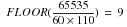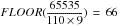# 15.00 - Examples - Teradata Database

prodname
vrm_release
15.00
category
User Guide
featnum
B035-1094-015K

### Examples

Consider the following PARTITION BY clause as an example of what happens with excess partitions. Assume that o_custkey has the INTEGER data type, o_orderdate has the DATE data type, and there are 8 columns defined for the table. Teradata Database defines 8-byte partitioning because the maximum combined partition number before adding excess partitions to a level is 462,000 ((8+2+1)*500*84), which is greater than the maximum combined partition number for 2‑byte partitioning, which is 65,335.

`      PARTITION BY (COLUMN,`
`                    RANGE_N(o_custkey BETWEEN 0`
`                                      AND     499999 `
`                                      EACH 1000),`
`                    RANGE_N(o_orderdate BETWEEN DATE '2003-01-01' `
`                                        AND     DATE '2009-12-31'`
`                                        EACH INTERVAL '1' MONTH) )`

The partitioning for this database object has the following characteristics.

• The number of column partitions defined for level 1 is 10, including two internal use column partitions and assuming a single column per partition.
• The maximum number of column partitions is 20, meaning that 10 additional column partitions could be added.

The maximum column partition number is 21.

• The number of row partitions defined for level 2 is 500.
• Teradata Database adds any excess partitions to level 2, so level 2 has a maximum of 5,228,668,955,133,092 row partitions.

The default for level 2 is `ADD 5228668955132592`.

• The number of row partitions defined for level 3 is 84, which is the same number of partitions defined for level 3.
• Because this is not the first row partitioning level that does not specify an ADD option, the default is `ADD 0`.

The implication of all this is that the partitioning specified by the preceding PARTITION BY clause is equivalent to the following PARTITION BY clause.

`     PARTITION BY (COLUMN ADD 10,`
`                   RANGE_N(o_custkey BETWEEN 0 `
`                                     AND     499999 `
`                                     EACH 1000) `
`                   ADD 5228668955132592,`
`                   RANGE_N(o_orderdate BETWEEN DATE '2003-01-01' `
`                                       AND     DATE '2009-12-31'`
`                                       EACH INTERVAL '1' MONTH) `
`                   ADD 0 )`

The adjusted maximum combined partition number is 9,223,372,036,854,774,288.

The following example is a full CREATE TABLE request that creates the column‑partitioned table named t1.

`     CREATE TABLE t1 (`
`       a01 INTEGER, a02 INTEGER, a03 INTEGER, a04 INTEGER, a05 INTEGER,`
`       a06 INTEGER, a07 INTEGER, a08 INTEGER, a09 INTEGER, a10 INTEGER,`
`       a11 INTEGER, a12 INTEGER, a13 INTEGER, a14 INTEGER, a15 INTEGER,`
`       a16 INTEGER, a17 INTEGER, a18 INTEGER, a19 INTEGER, a20 INTEGER,`
`       a21 INTEGER, a22 INTEGER, a23 INTEGER, a24 INTEGER, a25 INTEGER,`
`       a26 INTEGER, a27 INTEGER, a28 INTEGER, a29 INTEGER, a30 INTEGER,`
`       a31 INTEGER, a32 INTEGER, a33 INTEGER, a34 INTEGER, a35 INTEGER,`
`       a36 INTEGER, a37 INTEGER, a38 INTEGER, a39 INTEGER, a40 INTEGER,`
`       a41 INTEGER, a42 INTEGER, a43 INTEGER, a44 INTEGER, a45 INTEGER,`
`       a46 INTEGER, a47 INTEGER, a48 INTEGER, a49 INTEGER, a50 INTEGER,`
`       a51 INTEGER, a52 INTEGER, a53 INTEGER, a54 INTEGER, a55 INTEGER,`
`       a56 INTEGER, a57 INTEGER, a58 INTEGER, a59 INTEGER, a60 INTEGER,`
`       a61 INTEGER, a62 INTEGER, a63 INTEGER, a64 INTEGER, a65 INTEGER,`
`       a66 INTEGER, a67 INTEGER, a68 INTEGER, a69 INTEGER, a70 INTEGER,`
`       a71 INTEGER, a72 INTEGER, a73 INTEGER, a74 INTEGER, a75 INTEGER,`
`       a76 INTEGER, a77 INTEGER, a78 INTEGER, a79 INTEGER, a80 INTEGER,`
`       a81 INTEGER, a82 INTEGER, a83 INTEGER, a84 INTEGER, a85 INTEGER,`
`       a86 INTEGER, a87 INTEGER, a88 INTEGER, a89 INTEGER, a90 INTEGER,`
`       a91 INTEGER, a92 INTEGER, a93 INTEGER, a94 INTEGER, a95 INTEGER,`
`       a96 INTEGER, a97 INTEGER)`
`     NO PRIMARY INDEX`
`     PARTITION BY (RANGE_N(a2 BETWEEN 1 `
`                              AND    48 `
`                              EACH 1, `
`                   NO RANGE, UNKNOWN) `
`                   ADD 10,`
`                   COLUMN, `
`                   RANGE_N(a3 BETWEEN 1 `
`                              AND    50 `
`                              EACH 10));`

The partitioning for this table has the following characteristics.

• The number of partitions defined for level 1 is 50 and, initially, the maximum number of partitions and the maximum partition number for this level is (50+10) = 60 because there is an ADD 10 clause for this level.
• The number of partitions defined for level 2 is 99: 97 user-specified partitions plus 2 for internal use.
• Because there is a level of row partitioning without an ADD clause, the column partitioning level has a default of `ADD 10`, so the maximum number of partitions for this level is 109, with a maximum column partition number of 110.

• The number of partitions defined for level 3 is 5. Because there is no ADD clause specified for this level and it is the first row partitioning level without an ADD clause, assuming a default of ADD 0, the maximum combined partition number before adding excess partitions to a level is (60*110*5), or 33,000, which is not greater than 65,535, so this partitioning consumes 2 bytes in the row header.
• The actual maximum number of partitions for this level is the largest number that would not cause the maximum combined partition number to exceed 65,535. This value is. Therefore, this level has a default of `ADD 4`, and the maximum number of partitions for the level is 9.

The adjusted maximum combined partition number for the table is (60*110*9), or 59,400.

Any remaining excess partitions can be added to level 1, meaning that the maximum number of partitions for level 1 can be increased such that the maximum combined partition number does not exceed 65,535.

This value isand the `ADD 10` clause for level 1 can be replaced by `ADD 16`.

The implication of all this is that the partitioning specified by the preceding CREATE TABLE request is equivalent to the following CREATE TABLE request.

`     CREATE TABLE t1 (`
`       a01 INTEGER, a02 INTEGER, a03 INTEGER, a04 INTEGER, a05 INTEGER,`
`       a06 INTEGER, a07 INTEGER, a08 INTEGER, a09 INTEGER, a10 INTEGER,`
`       a11 INTEGER, a12 INTEGER, a13 INTEGER, a14 INTEGER, a15 INTEGER,`
`       a16 INTEGER, a17 INTEGER, a18 INTEGER, a19 INTEGER, a20 INTEGER,`
`       a21 INTEGER, a22 INTEGER, a23 INTEGER, a24 INTEGER, a25 INTEGER,`
`       a26 INTEGER, a27 INTEGER, a28 INTEGER, a29 INTEGER, a30 INTEGER,`
`       a31 INTEGER, a32 INTEGER, a33 INTEGER, a34 INTEGER, a35 INTEGER,`
`       a36 INTEGER, a37 INTEGER, a38 INTEGER, a39 INTEGER, a40 INTEGER,`
`       a41 INTEGER, a42 INTEGER, a43 INTEGER, a44 INTEGER, a45 INTEGER,`
`       a46 INTEGER, a47 INTEGER, a48 INTEGER, a49 INTEGER, a50 INTEGER,`
`       a51 INTEGER, a52 INTEGER, a53 INTEGER, a54 INTEGER, a55 INTEGER,`
`       a56 INTEGER, a57 INTEGER, a58 INTEGER, a59 INTEGER, a60 INTEGER,`
`       a61 INTEGER, a62 INTEGER, a63 INTEGER, a64 INTEGER, a65 INTEGER,`
`       a66 INTEGER, a67 INTEGER, a68 INTEGER, a69 INTEGER, a70 INTEGER,`
`       a71 INTEGER, a72 INTEGER, a73 INTEGER, a74 INTEGER, a75 INTEGER,`
`       a76 INTEGER, a77 INTEGER, a78 INTEGER, a79 INTEGER, a80 INTEGER,`
`       a81 INTEGER, a82 INTEGER, a83 INTEGER, a84 INTEGER, a85 INTEGER,`
`       a86 INTEGER, a87 INTEGER, a88 INTEGER, a89 INTEGER, a90 INTEGER,`
`       a91 INTEGER, a92 INTEGER, a93 INTEGER, a94 INTEGER, a95 INTEGER,`
`       a96 INTEGER, a97 INTEGER)`
`     NO PRIMARY INDEX`
`     PARTITION BY (RANGE_N(a2 BETWEEN 1 `
`                              AND    48 `
`                              EACH 1, `
`                   NO RANGE, UNKNOWN) `
`                   ADD 16,`
`                   COLUMN ADD 10, `
`                   RANGE_N(a3 BETWEEN 1 `
`                              AND    50 `
`                              EACH 10) `
`                   ADD 4);`

Now the maximum combined partition number is (66*110*9), or 65,340. The maximum number of combined partitions is (66*109*9), or 64,746. The number of combined partitions is (50*99*5), or 24,750 and you can alter the table to add additional partitions to each of the partitioning levels.